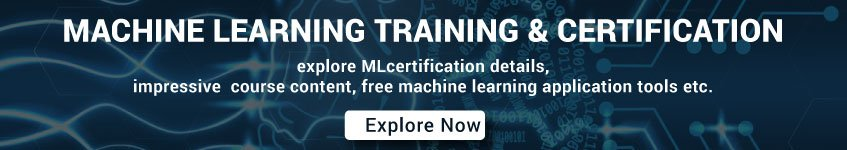# Machine Learning Interview Questions and Answers Set 1

1. What is machine learning?

In answering this question, try to show you understand of the broad applications of machine learning, as well as how it fits into AI. Put it into your own words, but convey your understanding that machine learning is a form of AI that automates data analysis to enable computers to learn and adapt through experience to do specific tasks without explicit programming

2. What is candidate sampling in machine learning?

A training-time optimization in which a probability is calculated for all the positive labels, using, for example, softmax, but only for a random sample of negative labels. For example, if we have an example labeled beagle and dog candidate sampling computes the predicted probabilities and corresponding loss terms for the beagle and dog class outputs in addition to a random subset of the remaining classes (cat, lollipop, fence).

3. Mention the difference between Data Mining and Machine learning?

Machine learning relates to the study, design, and development of the algorithms that give computers the capability to learn without being explicitly programmed.  While data mining can be defined as the process by which the unstructured data tries to extract knowledge or unknown interesting patterns.  During this processing machine, learning algorithms are used.

4. What is A/B testing in Machine Learning?

A statistical way of comparing two (or more) techniques, typically an incumbent against a new rival. A/B testing aims to determine not only which technique performs better but also to understand whether the difference is statistically significant. A/B testing usually considers only two techniques using one measurement, but it can be applied to any finite number of techniques and measures.

5. Explain How We Can Capture The Correlation Between Continuous And Categorical Variable?

Yes, it is possible by using ANCOVA technique. It stands for Analysis of Covariance.

It is used to calculate the association between continuous and categorical variables.

6. What is ‘Overfitting’ in Machine learning?

In machine learning, when a statistical model describes random error or noise instead of the underlying relationship ‘overfitting’ occurs.  When a model is excessively complex, overfitting is normally observed, because of having too many parameters with respect to the number of training data types. The model exhibits poor performance which has been overfitted.

7. Why does overfitting happen?

The possibility of overfitting exists as the criteria used for training the model is not the same as the criteria used to judge the efficacy of a model.

8. How can you avoid overfitting?

By using a lot of data overfitting can be avoided, overfitting happens relatively as you have a small dataset, and you try to learn from it. But if you have a small database and you are forced to come with a model based on that. In such a situation, you can use a technique known as cross-validation. In this method the dataset splits into two section, testing and training datasets, the testing dataset will only test the model while, in the training dataset, the data points will come up with the model.

In this technique,  a model is usually given a dataset of a known data on which training (training data set) is run and a dataset of unknown data against which the model is tested. The idea of cross-validation is to define a dataset to “test” the model in the training phase.

9. Explain Principal Component Analysis (PCA).

PCA is a dimensionality-reduction technique which mathematically transforms a set of correlated variables into a smaller set of uncorrelated variables called principal components.

10. What value do you optimize when using a support vector machine (SVM)?

For a linear function, SVM optimizes the product of input vectors as well as the coefficients. In other words, the algorithm with the linear function can be restructured into a dot-product.